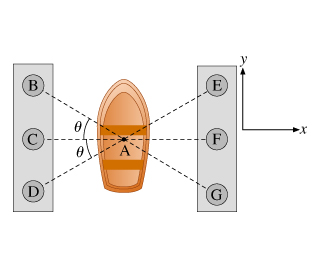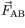# Problem: A boat owner pulls her boat into the dock shown, where there are six capstans to which to tie the boat. She has three ropes. She can tie the boat from the boat's center (A) to any of the capstans (B through G) along the dotted arrows shown.Suppose the owner has tied three ropes: one rope runs to A from B, another to A from D, and a final rope from A to F. The ropes are tied such that FAB = FAD .The following notation is used in this problem: When a question refers to, for example, , this quantity is taken to mean the force acting on the boat due to the rope running to A from B, while FAB is the magnitude of that force.What is the magnitude of the force provided by the third rope, in terms of θ?A. FABCos(θ)B. 2FABCos(θ)C. 2FABSin(θ)D.FABSin(θ)

###### FREE Expert Solution

Equilibrium condition along the x-axis:

$\overline{){\mathbf{\Sigma }}{\mathbf{F}}{\mathbf{=}}{\mathbf{0}}}$

Fx = F cos θ

FAF - FABx - FADx = 0

100% (95 ratings)###### Problem Details

A boat owner pulls her boat into the dock shown, where there are six capstans to which to tie the boat. She has three ropes. She can tie the boat from the boat's center (A) to any of the capstans (B through G) along the dotted arrows shown.
Suppose the owner has tied three ropes: one rope runs to A from B, another to A from D, and a final rope from A to F. The ropes are tied such that FAB = FAD .The following notation is used in this problem: When a question refers to, for example,, this quantity is taken to mean the force acting on the boat due to the rope running to A from B, while FAB is the magnitude of that force.

What is the magnitude of the force provided by the third rope, in terms of θ?

A. FABCos(θ)

B. 2FABCos(θ)

C. 2FABSin(θ)

D.FABSin(θ)